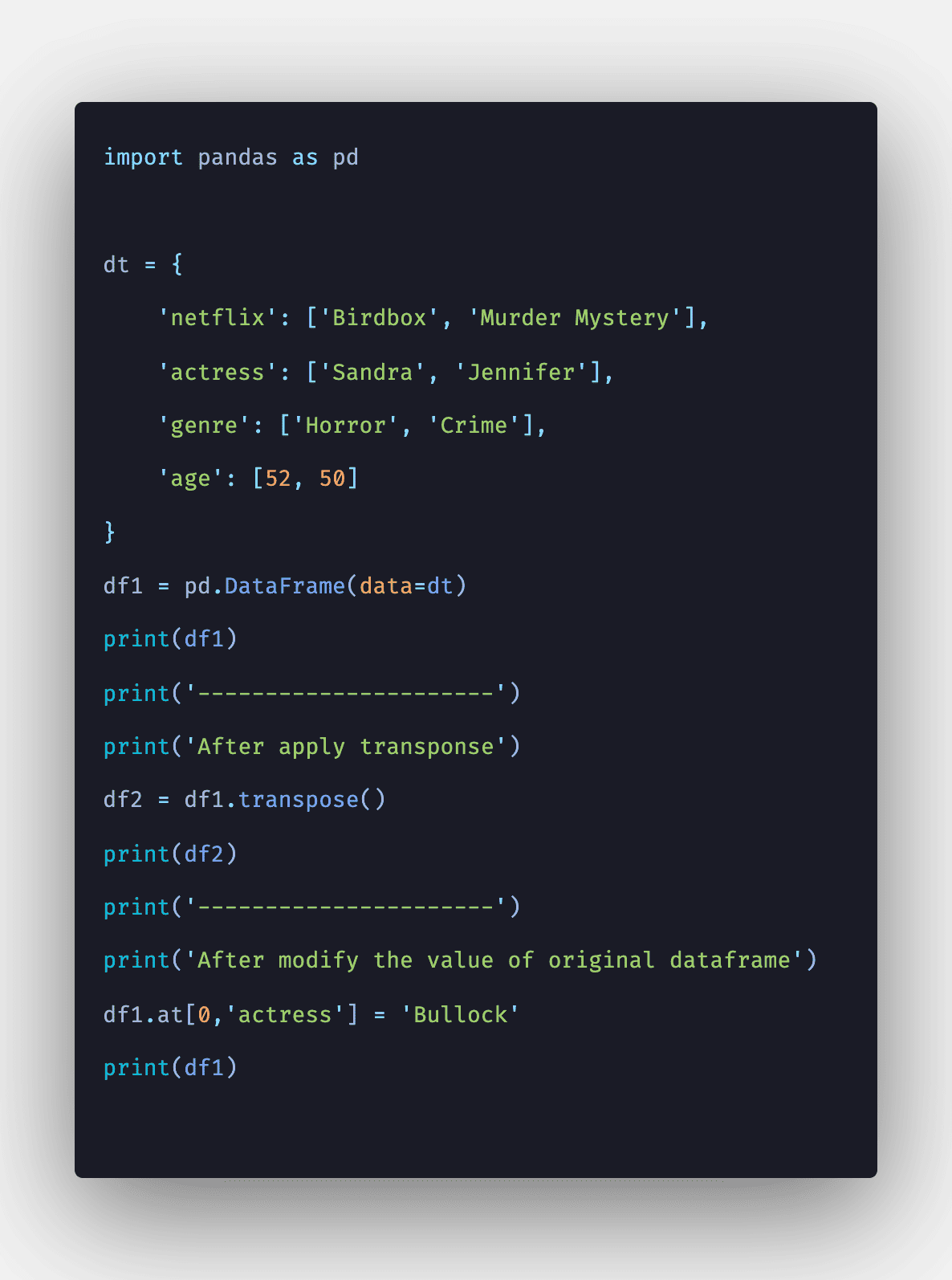# How to Transpose Matrix in Python

0
1293Pandas DataFrame is a two-dimensional, size-mutable, potentially complex tabular data structure with labeled axes (rows and columns).

## Pandas transpose

Pandas DataFrame.transpose() is a library function that transpose index and columns. The transpose reflects the DataFrame over its main diagonal by writing rows as columns and vice-versa. Use the T attribute or the transpose() method to swap (= transpose) the rows and columns of DataFrame.

Neither method changes an original object but returns the new object with the rows and columns swapped (= transposed object).

Here, one thing to note is that depending on the data type dtype of each column, the view is created instead of the copy, and changing the value of one of the original and transposed objects will change the other.

### Syntax

`DataFrame.transpose(self, *args, **kwargs)`

### Parameters

*args: tuple, optional

Accepted for compatibility with NumPy.

copy: bool, default False

Whether to copy a data after transposing, even for DataFrames with the single dtype. Note that the copy is always required for mixed dtype DataFrames, or DataFrames with any extension types.

### Return Value

The transposed DataFrame. Transposing the DataFrame with mixed dtypes will result in the homogeneous DataFrame with an object dtype. In such a case, a copy of the data is always made.

### Step 1: Define DataFrame

Let’s define a DataFrame using Pandas.

```# app.py

import pandas as pd

dt = {
'Stranger Things': ['Mike', 'Eleven'],
'Money Heist': ['Professor', 'Tokyo']
}

df1 = pd.DataFrame(data=dt)
print(df1)```

#### Output

```python3 app.py
Stranger Things Money Heist
0            Mike   Professor
1          Eleven       Tokyo```

In the above output. and are index, and Stanger Things and Money Heist are columns.

### Step 2: pandas.DataFrame.T

We can transpose the DataFrame using T. The property T is an accessor to the method transpose(). So let’s transpose the dataframe using T. See the following code.

```# app.py

import pandas as pd

dt = {
'Stranger Things': ['Mike', 'Eleven'],
'Money Heist': ['Professor', 'Tokyo']
}

df1 = pd.DataFrame(data=dt)
print(df1)
print('----------------------')
print('After apply transponse')
tdf1 = df1.T
print(tdf1)```

#### Output

```python3 app.py
Stranger Things Money Heist
0            Mike   Professor
1          Eleven       Tokyo
----------------------
After apply transponse
0       1
Stranger Things       Mike  Eleven
Money Heist      Professor   Tokyo```

After applying transpose, the rows become columns, and columns become rows in DataFrame.

So, it returns the transposed DataFrame. If you have learned Matrix in college, you are pretty familiar with the Transpose of Matrix.

## Pandas.DataFrame.transpose()

In the above example, we have used T, but you can also use the transpose() method.

```import pandas as pd

dt = {
'Stranger Things': ['Mike', 'Eleven'],
'Money Heist': ['Professor', 'Tokyo']
}

df1 = pd.DataFrame(data=dt)
print(df1)
print('----------------------')
print('After apply transponse')
tdf1 = df1.transpose()
print(tdf1)```

#### Output

```python3 app.py
Stranger Things Money Heist
0            Mike   Professor
1          Eleven       Tokyo
----------------------
After apply transponse
0       1
Stranger Things       Mike  Eleven
Money Heist      Professor   Tokyo```

## Pandas transpose: Change the original object itself

The parameter, like inplace that changes the original object itself, is not provided. If you do not want to create a new object, you can assign it to the original object itself.

See the following code.

```# app.py

import pandas as pd

dt = {
'Stranger Things': ['Mike', 'Eleven'],
'Money Heist': ['Professor', 'Tokyo']
}

df1 = pd.DataFrame(data=dt)
print(df1)
print('----------------------')
print('After apply transponse')
df1 = df1.transpose()
print(df1)```

#### Output

```python3 app.py
Stranger Things Money Heist
0            Mike   Professor
1          Eleven       Tokyo
----------------------
After apply transponse
0       1
Stranger Things       Mike  Eleven
Money Heist      Professor   Tokyo```

## Pandas transpose: Non-square DataFrame with mixed dtypes

Let’s take an example in which we will create a non-square DataFrame.

```# app.py

import pandas as pd

dt = {
'netflix': ['Birdbox', 'Murder Mystery'],
'actress': ['Sandra', 'Jennifer'],
'genre': ['Horror', 'Crime'],
'age': [52, 50]
}
df1 = pd.DataFrame(data=dt)
print(df1)
print('----------------------')
print('After apply transponse')
df1 = df1.transpose()
print(df1)```

#### Output

```python3 app.py
netflix   actress   genre  age
0         Birdbox    Sandra  Horror   52
1  Murder Mystery  Jennifer   Crime   50
----------------------
After apply transponse
0               1
netflix  Birdbox  Murder Mystery
actress   Sandra        Jennifer
genre     Horror           Crime
age           52              50```

## Pandas transpose: View and copy

If the data type dtype is different for each column, T or transpose() generates a copy. If you change the value of one of the original and the transposed object, the other remains the same.

See the following code.

```# app.py

import pandas as pd

dt = {
'netflix': ['Birdbox', 'Murder Mystery'],
'actress': ['Sandra', 'Jennifer'],
'genre': ['Horror', 'Crime'],
'age': [52, 50]
}
df1 = pd.DataFrame(data=dt)
print(df1)
print('----------------------')
print('After apply transponse')
df2 = df1.transpose()
print(df2)
print('----------------------')
print('After modify the value of original dataframe')
df1.at[0,'actress'] = 'Bullock'
print(df1)
```

#### Output

```python3 app.py
netflix   actress   genre  age
0         Birdbox    Sandra  Horror   52
1  Murder Mystery  Jennifer   Crime   50
----------------------
After apply transponse
0               1
netflix  Birdbox  Murder Mystery
actress   Sandra        Jennifer
genre     Horror           Crime
age           52              50
----------------------
After modify the value of original dataframe
netflix   actress   genre  age
0         Birdbox   Bullock  Horror   52
1  Murder Mystery  Jennifer   Crime   50```

From the output, you can see that the actress value has been modified from Sandra to Bullock.

## Conclusion

To find the transpose of the Matrix, you can use the Pandas library and create a DataFrame and use the transpose() function to find the transpose of the given DataFrame.